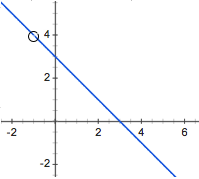Home > APCALC > Chapter 1 > Lesson 1.4.3 > Problem1-173

1-173.

If $f(x)=\frac{x^2-2x-3}{x-3}$ and $g(x)=-x+2$, write an equation for $h(x)=f(g(x))$.

$h(x)=f(g(x))=f(-x+2)=\frac{(-x+2)^{2}-2(-x+2)-3)}{(-x+2)-3}$

$-\frac{(x-3)(x+1)}{x+1}=-x+3\text{ unless }x= -1$

1. Graph $y=h(x)$ and write its domain in set notation.$D=x:x\ne-1$

2. Write an equation the end-behavior function of $h$.

The end behavior of $h(x)$ will describe what $h(x)$ looks like in the end (as $x→∞$ and as $x→-∞$). Holes and vertical asymptotes are ignored. Observe the graph in part (a). Notice that the graph of $h(x)$ is almost linear. What is the equation of this line? That line, by the way, is $b(x)$, the end behavior of $h(x)$.